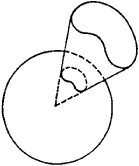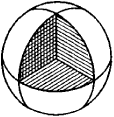# Solid Angle

(redirected from Solid angle measure)
Also found in: Dictionary, Thesaurus.

## solid angle

[′säl·əd ′aŋ·gəl]
(mathematics)
A surface formed by all rays joining a point to a closed curve.
McGraw-Hill Dictionary of Scientific & Technical Terms, 6E, Copyright © 2003 by The McGraw-Hill Companies, Inc.
The following article is from The Great Soviet Encyclopedia (1979). It might be outdated or ideologically biased.

## Solid Angle

a surface formed by rays having a common origin and passing through a closed curve (Figure 1). Sometimes, particularly in Soviet usage, the term “solid angle” is applied to the portion of space bounded by such a surface. Trihedral and polyhedral angles are special cases of solid angles.Figure 1

A measure of the solid angle subtended at a given point by a surface S is provided by the ratio AIR2. Here, A is the area of the portion of a sphere, with center at the given point, that is cut by a conical surface with vertex at the point and with the perimeter of S as a directrix, and R is the radius of the sphere. Clearly, the measure of a solid angle is an abstract number. For example, the measure of a solid angle that encloses an octant, that is, oneeighth of space, is the number 4π R2/8 R2 = π/2 (Figure 2).Figure 2

The unit of measure of a solid angle is called the steradian. It is equal to the solid angle subtended at the center of a sphere of unit radius by a portion of the sphere’s surface that is of unit area. The total solid angle about a point is equal to 4π steradians.